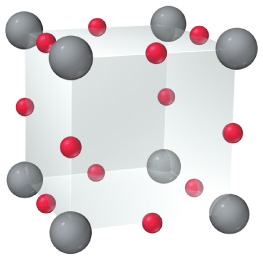# Problem: An oxide of rhenium crystallizes with the unit cell depicted here (where rhenium = gray and oxygen = red).What is the formula of the oxide?

###### FREE Expert Solution

Recall that for a unit cell

**Atoms on the corner will have 1/8 of its volume on the unit cell

**Atoms on the cube face will have 1/4 of its volume on the unit cell

This means that:

93% (313 ratings)###### Problem Details
An oxide of rhenium crystallizes with the unit cell depicted here (where rhenium = gray and oxygen = red).

What is the formula of the oxide?

Frequently Asked Questions

What scientific concept do you need to know in order to solve this problem?

Our tutors have indicated that to solve this problem you will need to apply the Unit Cell concept. You can view video lessons to learn Unit Cell. Or if you need more Unit Cell practice, you can also practice Unit Cell practice problems.

What professor is this problem relevant for?

Based on our data, we think this problem is relevant for Professor Randles' class at UCF.